# Inverse problem and source analysis#

Estimate source activations, extract activations in labels, morph data between subjects etc.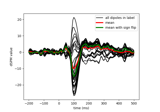Compute MNE-dSPM inverse solution on single epochs

Compute MNE-dSPM inverse solution on single epochs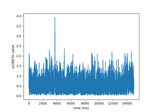Compute sLORETA inverse solution on raw data

Compute sLORETA inverse solution on raw data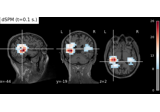Compute MNE-dSPM inverse solution on evoked data in volume source space

Compute MNE-dSPM inverse solution on evoked data in volume source space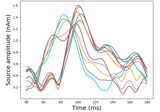Source localization with a custom inverse solver

Source localization with a custom inverse solver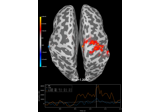Compute source level time-frequency timecourses using a DICS beamformer

Compute source level time-frequency timecourses using a DICS beamformer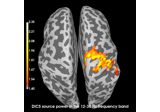Compute source power using DICS beamformer

Compute source power using DICS beamformer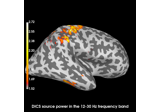Compute evoked ERS source power using DICS, LCMV beamformer, and dSPM

Compute evoked ERS source power using DICS, LCMV beamformer, and dSPM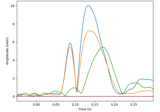Compute a sparse inverse solution using the Gamma-MAP empirical Bayesian method

Compute a sparse inverse solution using the Gamma-MAP empirical Bayesian method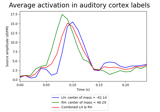Extracting time course from source_estimate object

Extracting time course from source_estimate object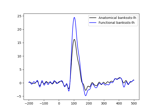Generate a functional label from source estimates

Generate a functional label from source estimates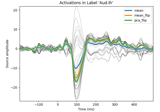Extracting the time series of activations in a label

Extracting the time series of activations in a label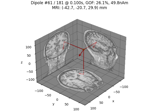Compute sparse inverse solution with mixed norm: MxNE and irMxNE

Compute sparse inverse solution with mixed norm: MxNE and irMxNE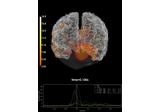Compute MNE inverse solution on evoked data with a mixed source space

Compute MNE inverse solution on evoked data with a mixed source space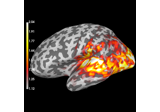Compute source power estimate by projecting the covariance with MNE

Compute source power estimate by projecting the covariance with MNE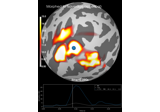Morph surface source estimate

Morph surface source estimate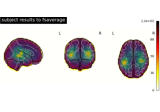Morph volumetric source estimate

Morph volumetric source estimate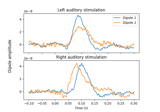Computing source timecourses with an XFit-like multi-dipole model

Computing source timecourses with an XFit-like multi-dipole model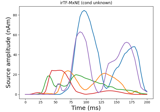Compute iterative reweighted TF-MxNE with multiscale time-frequency dictionary

Compute iterative reweighted TF-MxNE with multiscale time-frequency dictionary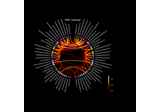Visualize source leakage among labels using a circular graph

Visualize source leakage among labels using a circular graph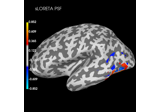Plot point-spread functions (PSFs) and cross-talk functions (CTFs)

Plot point-spread functions (PSFs) and cross-talk functions (CTFs)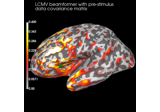Compute cross-talk functions for LCMV beamformers

Compute cross-talk functions for LCMV beamformers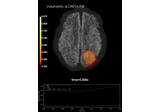Plot point-spread functions (PSFs) for a volume

Plot point-spread functions (PSFs) for a volume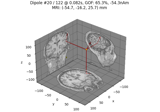Compute Rap-Music on evoked data

Compute Rap-Music on evoked data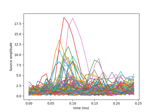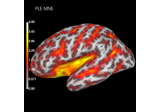Compute spatial resolution metrics in source space

Compute spatial resolution metrics in source space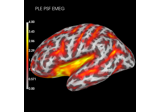Compute spatial resolution metrics to compare MEG with EEG+MEG

Compute spatial resolution metrics to compare MEG with EEG+MEG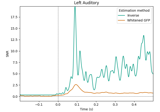Estimate data SNR using an inverse

Estimate data SNR using an inverse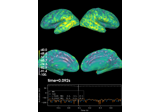Computing source space SNR

Computing source space SNR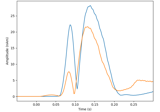Compute MxNE with time-frequency sparse prior

Compute MxNE with time-frequency sparse priorCompute Trap-Music on evoked data

Compute Trap-Music on evoked data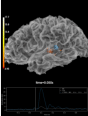Plotting the full vector-valued MNE solution

Plotting the full vector-valued MNE solution